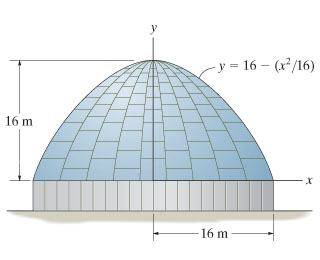# Surface area of a dome-ish roof

## Homework Statement

Determine the surface area of the roof of the structure if it is formed by rotating the parabola about the axis.## Homework Equations

$SA=\int _0^{16}{2\pi\left ( 4 \sqrt{16-y} \right ) dy}$ (???)

## The Attempt at a Solution

$SA=\left [ -\frac{16}{3}\pi\left ( 16-y \right )^{\frac{3}{2}}\right ]_{0}^{16}$
$SA=1072.33$

So, 1072.33 m^2?

That's what I get.

Well according to page 78 of http://www.slideshare.net/mrsbeth63/engineering-mechanics-statics-rc-hibbeler-12th-edition-complete-solutions-ch-9" [Broken], the answer is 1365 m^2. I kind of understand how they did it, I just want to know why my way doesn't work.

Last edited by a moderator:
Dick
Homework Helper
Well according to page 78 of http://www.slideshare.net/mrsbeth63/engineering-mechanics-statics-rc-hibbeler-12th-edition-complete-solutions-ch-9" [Broken], the answer is 1365 m^2. I kind of understand how they did it, I just want to know why my way doesn't work.

Because that's not a formula for surface area. You are integrating 2*pi*f(y). You want to integrate 2*pi*f(y)*sqrt(1+f'(y)^2).

Last edited by a moderator:
Because that's not a formula for surface area. You are integrating 2*pi*f(y). You want to integrate 2*pi*f(y)*sqrt(1+f'(y)^2).
Why not? How does adding up the circumferences not equal the surface area of the dome.

Dick
Homework Helper
Why not? How does adding up the circumferences not equal the surface area of the dome.

You are adding up infinitesimal surface areas. If you just use the circumference then you are assuming a cylinder is a good approximation to the cross sectional surface area. It's not. Try applying that to a cone. You'll get the wrong answer.

Last edited:
Why not? How does adding up the circumferences not equal the surface area of the dome.

surface area is only the outer shell of a solid, which is the SA. what you are doing is finding the area under the curve and multiplying it by 2pi

SA is the arc length (you can think of it as circumference) rotated around 2pi for this problem and the formula for arc length is √(1+[y']^2)

You are adding up infinitesimal surface areas. If you just use the circumference then you are assuming a cylinder is a good approximation to the cross sectional surface area. It's not. Try applying that to a cone. You'll get the wrong answer.
Okay thanks.

Calculus is stupid.